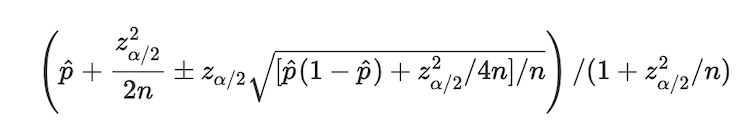# What does Top-Rated Mean in Reddit’s Ranking SystemThough Reddit’s comment sorting system has been in use for almost a decade, the fact is that I wasn’t so exposed to western internet community till 2013, it is still, a rather new thing to me.

Randall, the author of xkcd, also my favourite internet comics, wrote a blog explaining what the algorithm is. Meanwhile, a detailed version is introduced by Evan Miller.

In the latter, Evan gave two examples of two “wrong” ranking methods:

1. Score = positive - negative;
2. Score = positive / total.

To say they are “wrong”, does not mean that they are not possible to give a rough idea in all scenarios. But the reality is always complex, a rough idea is probably a synonym of “meaninglessness”. To be specific, the first case ignore the “ratio” part in the term of “highest rated”, that means a more controversial comment might exceed a quality post simply due to more people voting on it. The second case, however, ignores the scenario where the sample space is limited. For instance, we can hardly say that a 1 of 1 upvoted comment is better than that of 99 of 100 upvoted comment.

How Reddit deals with this problem is trying to reach a confident balance between positive proportion and small number of observations. Now suppose the following:

1. Each voting event is independent.
2. Each event can either be a positive or a negative.
3. The total number of votes is $n$, the number of positive votes is $k$, $\hat{p}=k/n$ is the positive proportion.

Now the idea is to first find each $\hat{p}$, then calculate the corresponding confidence intervals, and finally rank the items by their lower bounds of confidence intervals.

The perfect expression of these confidence intervals here is:In 1928, mathematician Edwin Bidwell Wilson developed this score interval above to estimate the successful (or positive, in our case) probability $\hat{p}$.

Here’s my R script to simulate a comment ranking situation possibly happening every day on Reddit.

library(dplyr)

Wilson <- function(n, k, alpha=0.95) {
phat <- k/n
score <- qnorm(1-(1-alpha)/2)
lbound <- (phat+score**2/(2*n)-score*sqrt((phat*(1-phat)+score**2/(4*n))/n))/(1+score**2/n)
return(lbound)
}

seed(2019)
x <- sample(1:500,200,replace=T)
y <- sample(1:500,200,replace=T)

rowwise() %>%
mutate(pos=min(x,y),total=max(x,y)) %>%
select(total, pos) %>%
mutate(Wilson=Wilson(total,pos)) %>%
arrange(desc(Wilson))


And the pseudo-results are:

# A tibble: 200 x 3
total   pos Wilson
<int> <int>  <dbl>
1   473   467  0.973
2   494   487  0.971
3   390   385  0.970
4   234   232  0.969
5   354   347  0.960
6   237   233  0.957
7   123   121  0.943
8   391   376  0.938
9   296   285  0.935
10   415   397  0.932
# ... with 190 more rows